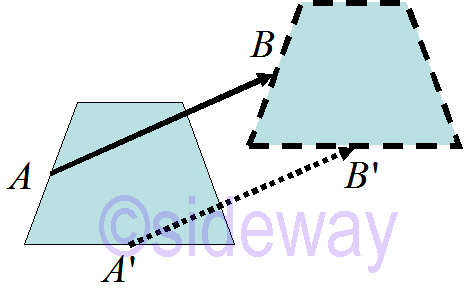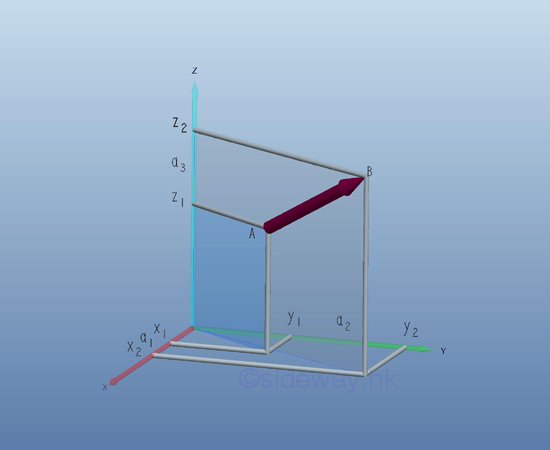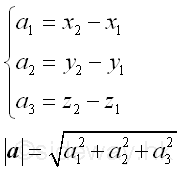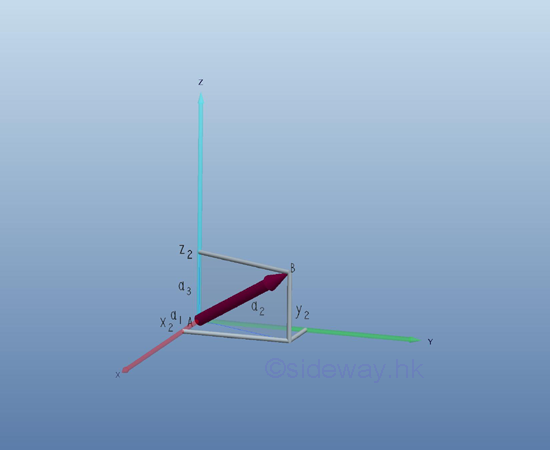output.to from Sideway
Algebra

Vector

Draft for Information Only

# Content

```  Vectors  Components of a Vector```

## Vectors

In practical applications, many quantities can be descibed by a magnitude completely in the form of size or number of units according to a scale. For examples, mass of a body, volume of a body, etc. These kinds of quantities are called scalars, or scalar quantites. However, there are also many quantities cannot be descibed by only one single number completely. For example, force of an applied load, velocity of a body. These kinds of quantities require another characteristic specification, a direction, to complete the description of a quantity. In general, these kinds of quantities can be represeneted graphically by directed line segment, arrow, with which the direction is indicated by the arrow cue pointing and the magnitude is represented by the total length of the arrow. As quantities characterized by both direction and magnitude can be represented by a directed line segment, these kinds of quantities are called vectors, or vector quantities.One practical application example is the translation of a body. The translation motion of the body is charactered by both the linear displacement and the direction of the movement. Similarly the translation can be graphically represented by a directed line segment linking the original reference position A of a point  on the body as the initial point and the final reference position B of the same point on the body after translation as the terminal point. Since the motion of each point on the body is the same, a group of directed line segments of the same length and same direction can be constructed for every point of the body. Therefore graphically a vector is a directed line segment used to represent the specified quantity with the length of the directed line segment is equal to the length of the vector and the direction of the directed line segment is equal to the direction of the vector. Since two equal directed line segments imply two equal quantities, two vector quantities are equal if and only if both vectors have the same length and  the same direction. the directed arrow is also called the sense of the vector. The length of a vector is also called the magnitude or the Euclidean norm of the vector.

Mathematically, a vector is usually denoted by a lowercase boldfaced letter, e.g 𝐚 or a little arrow overhead letter a. And the length of a vector is usually denoted by the absolute value symbol e.g. |𝐚|. A vector with length equal to 1 is called a unit vector.

## Components of a Vector

Since a point can be considered as a geometric object in a three dimensional space, a point can be represented by the coordinates of a coordinate system such that a vector can be expressed algebraically. The most common system for a three dimensional space is the rectangular coordinate system called Cartesian coordinate system with three mutually perpendicular straight axes of same scale. The initial point A and terminal point B of a vector 𝐚 can be represented by coordinates A(x1,y1,z1) and B(x2,y2,z2). And the geometry of the directed line segment corresponding to the vector can be specified by the numbers a1=x2-x1, a2=y2-y1, and a3=z2-z1. These numbers are called the components of the vector 𝐚 with respect to the corresponding Cartesian coordinate system because vector 𝐚 can be obtained from these components.Geometrically, the length |𝐚| of the vector 𝐚 can be determined by the Pythagorean theorem.Physically, a vector can be translated arbitrarily to indicate the quantity of a point that with equal quantity, the terminal point of the vector can be determined uniquely once the initial point of the vector is fixed. If the initial point of a vector is located at the origin, the components of the vector are then equal to the coordinate of the terminal point. The vector with initial point at the origin is called the position vector 𝐫 of the terminal point with respect to the corresponding Cartesian coordinate system. As the components a1, a2, and a3 of vector 𝐚 are derived from the end points of the vector by subtracting the coordinates of initial point from the coordinates of the terminal point, the components of vector are independent of the choice of the initial point of the vector and is dependent on the manitude and direction of the vector only. Therefore, any vector in three dimensional space can be represented by a position vector by translating the initial point of the vector to the origin. And for a specifed Cartesian coordinate system,  each vector in space can be determined by the ordered triple components of the vector with respect to the corresponding Cartesian coordinate system of one-to-one relation. In other words, two vectors 𝐚 and 𝐛 are equal if and only if the corresponding component of two vectors are equal. That is a1=b1, a2=b2, and a3=b3As both the magnitude and the direction of the directed line segment of the corresponding vector can be obtained from the components of the corresponding vector, the geometry of the directed line segment in three dimensional space can also be determined by the ordered triple components of the vector with respect to the corresponding Cartesian coordinate system of one-to-one relation.

However, the vector representation of quantity with magnitude and direction is dependent on the choice of coordinate system.

©sidewayReferences

1. B. Joseph, 1978, University Mathematics: A Textbook for Students of Science & Engineering, Blackie & Son Limited, HongKong
2. Wheatstone, C., 1854, On the Formation of Powers from Arithmetical Progressions, Proceedings of The Royal Society of London, Vol 7, p145-151,, London
3. Stroud, K.A., 2001, Engineering Mathematics, Industrial Press, Inc, NY
4. Coolidge, J.L., 1949, The Story of The Binomial Theorem, The American Mathematical Monthly, Vol 56, No.3, Mar, pp147-157ID: 130800174 Last Updated: 2013/8/21 Revision: Ref:Home (5)

Business

Management

HBR (3)

Information

Recreation

Hobbies (7)

Culture

Chinese (1097)

English (336)

Reference (66)

Computer

Hardware (149)

Software

Application (187)

Digitization (24)

Numeric (19)

Programming

Web (644)CSS (SC)

ASP.NET (SC)

HTML

Knowledge Base

Common Color (SC)

Html 401 Special (SC)

OS (389)MS Windows

Windows10 (SC)

.NET Framework (SC)

DeskTop (7)

Knowledge

Mathematics

Formulas (8)

Number Theory (206)

Algebra (20)

Trigonometry (18)

Geometry (18)

Calculus (67)

Complex Analysis (21)

Engineering

Tables (8)

Mechanical

Mechanics (1)

Rigid Bodies

Statics (92)

Dynamics (37)

Fluid (5)

Control

Acoustics (19)

Biology (1)

Geography (1)

Latest Updated Links

Copyright © 2000-2019 Sideway . All rights reserved Disclaimers last modified on 10 Feb 2019